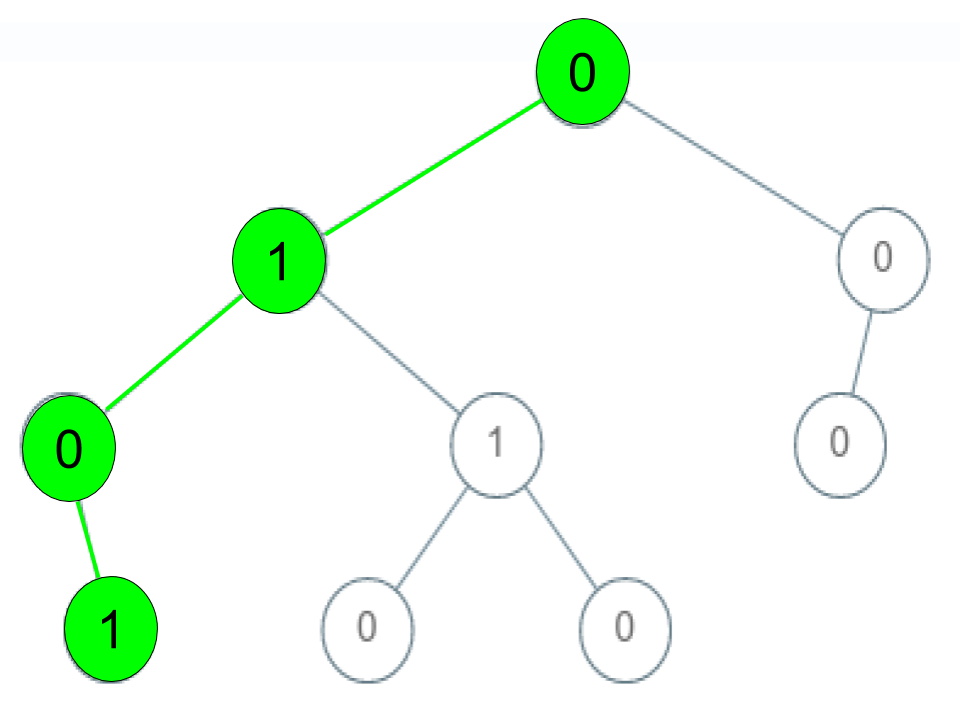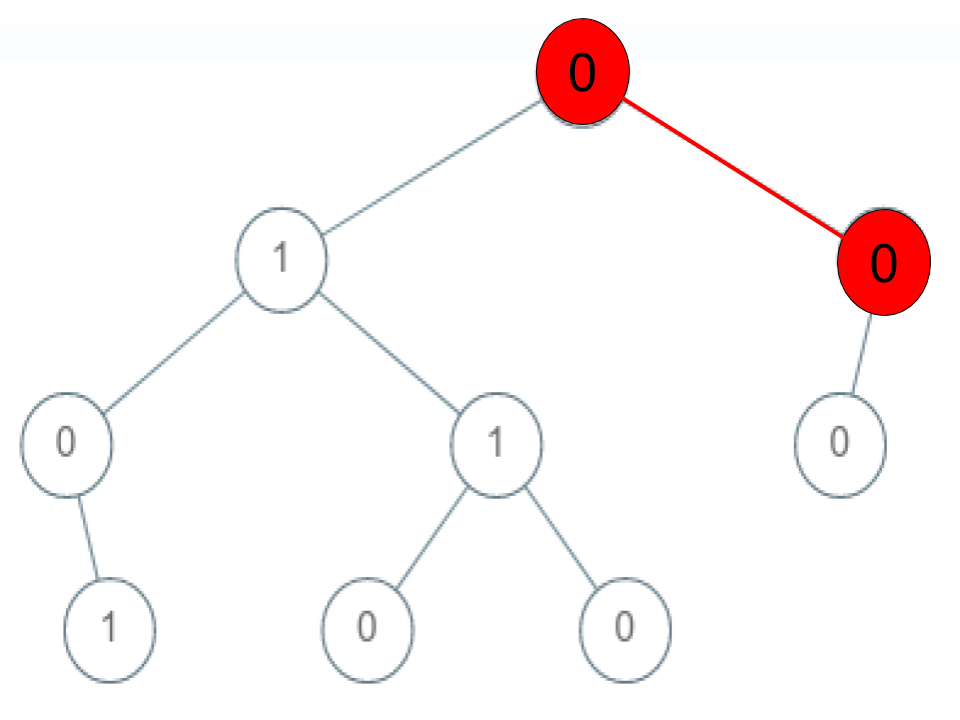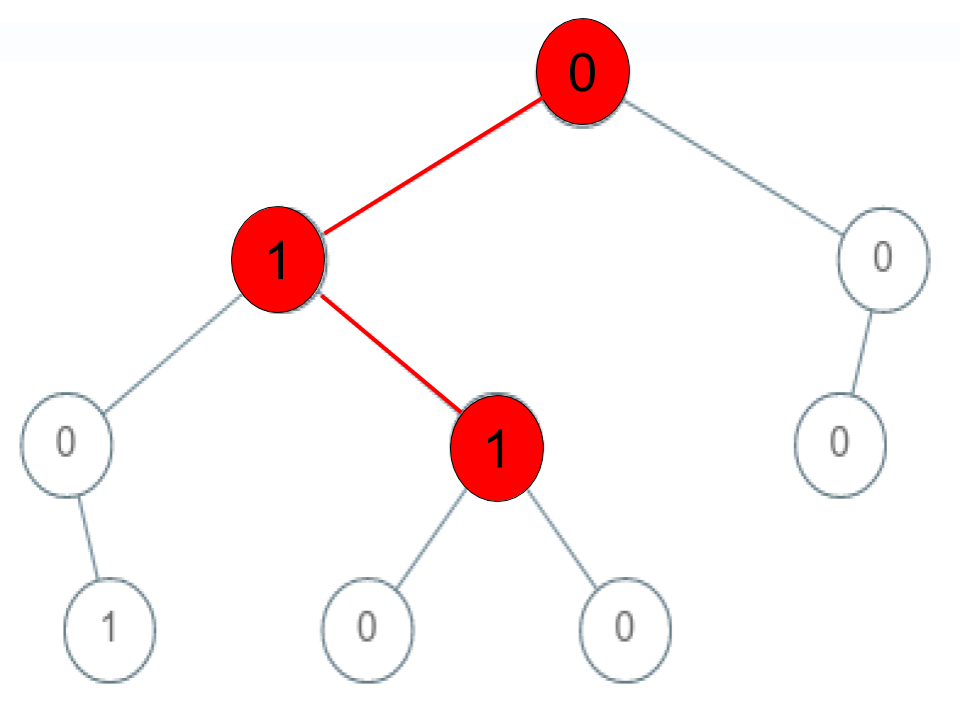Given a binary tree where each path going from the root to any leaf form a valid sequence, check if a given string is a valid sequence in such binary tree.

We get the given string from the concatenation of an array of integers arr and the concatenation of all values of the nodes along a path results in a sequence in the given binary tree.

Example 1:Input: root = [0,1,0,0,1,0,null,null,1,0,0], arr = [0,1,0,1] Output: true Explanation: The path 0 -> 1 -> 0 -> 1 is a valid sequence (green color in the figure). Other valid sequences are: 0 -> 1 -> 1 -> 0 0 -> 0 -> 0

Example 2:Input: root = [0,1,0,0,1,0,null,null,1,0,0], arr = [0,0,1] Output: false Explanation: The path 0 -> 0 -> 1 does not exist, therefore it is not even a sequence.

Example 3:Input: root = [0,1,0,0,1,0,null,null,1,0,0], arr = [0,1,1] Output: false Explanation: The path 0 -> 1 -> 1 is a sequence, but it is not a valid sequence.

Constraints:

• 1 <= arr.length <= 5000
• 0 <= arr[i] <= 9
• Each node’s value is between [0 – 9].
* Image Source : LeetCode

#### Solution

Its a tree traversal problem only, the twist here is to check if any path from root to leaf present which will match with input array.

###### Code

We encourage you to write a comment if you have a better solution or having any doubt on the above topic.